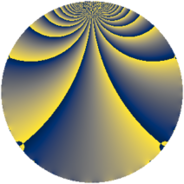# Properties

 Label 637.2.xLevel $637$ Weight $2$ Character orbit 637.x Rep. character $\chi_{637}(19,\cdot)$ Character field $\Q(\zeta_{12})$ Dimension $172$ Newform subspaces $3$ Sturm bound $130$ Trace bound $1$

# Related objects

## Defining parameters

 Level: $$N$$ $$=$$ $$637 = 7^{2} \cdot 13$$ Weight: $$k$$ $$=$$ $$2$$ Character orbit: $$[\chi]$$ $$=$$ 637.x (of order $$12$$ and degree $$4$$) Character conductor: $$\operatorname{cond}(\chi)$$ $$=$$ $$91$$ Character field: $$\Q(\zeta_{12})$$ Newform subspaces: $$3$$ Sturm bound: $$130$$ Trace bound: $$1$$ Distinguishing $$T_p$$: $$2$$

## Dimensions

The following table gives the dimensions of various subspaces of $$M_{2}(637, [\chi])$$.

Total New Old
Modular forms 292 204 88
Cusp forms 228 172 56
Eisenstein series 64 32 32

## Trace form

 $$172q + 2q^{2} + 6q^{4} + 6q^{5} - 12q^{6} - 20q^{8} - 140q^{9} + O(q^{10})$$ $$172q + 2q^{2} + 6q^{4} + 6q^{5} - 12q^{6} - 20q^{8} - 140q^{9} + 12q^{10} - 10q^{11} - 8q^{12} - 46q^{15} + 66q^{16} + 6q^{17} - 36q^{18} + 8q^{19} + 36q^{20} - 4q^{22} + 6q^{23} - 12q^{24} - 24q^{26} - 4q^{29} + 38q^{31} + 44q^{32} - 18q^{33} - 12q^{34} - 138q^{36} - 12q^{37} - 96q^{39} - 48q^{40} - 18q^{41} - 26q^{44} - 12q^{45} - 26q^{46} + 42q^{47} - 12q^{48} - 90q^{50} + 120q^{51} + 28q^{52} - 8q^{53} + 30q^{54} + 6q^{55} + 16q^{57} - 62q^{58} + 6q^{59} + 108q^{60} + 36q^{62} - 6q^{65} - 66q^{66} + 60q^{67} - 30q^{68} - 42q^{69} + 10q^{71} + 94q^{72} - 14q^{73} + 74q^{74} + 20q^{75} - 52q^{76} + 22q^{78} - 20q^{79} - 12q^{80} - 28q^{81} + 108q^{82} + 66q^{83} + 102q^{85} + 14q^{86} - 42q^{87} + 30q^{89} + 72q^{90} + 188q^{92} + 118q^{93} - 18q^{95} - 18q^{96} - 62q^{97} + 48q^{99} + O(q^{100})$$

## Decomposition of $$S_{2}^{\mathrm{new}}(637, [\chi])$$ into newform subspaces

Label Dim. $$A$$ Field CM Traces $q$-expansion
$$a_2$$ $$a_3$$ $$a_5$$ $$a_7$$
637.2.x.a $$28$$ $$5.086$$ None $$-2$$ $$0$$ $$6$$ $$0$$
637.2.x.b $$32$$ $$5.086$$ None $$4$$ $$0$$ $$0$$ $$0$$
637.2.x.c $$112$$ $$5.086$$ None $$0$$ $$0$$ $$0$$ $$0$$

## Decomposition of $$S_{2}^{\mathrm{old}}(637, [\chi])$$ into lower level spaces

$$S_{2}^{\mathrm{old}}(637, [\chi]) \cong$$ $$S_{2}^{\mathrm{new}}(91, [\chi])$$$$^{\oplus 2}$$